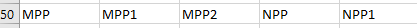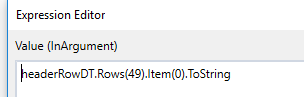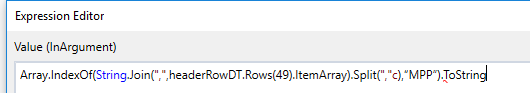# Get column index and row index for specific values

Hey everybody,

I have a huge excel file(s) with different sheets. Since the next sheets and next files can look slightly different from each other there is a common header in each file to find the correct column. So I only get the information in which row the common header is (e.g. row 50 in the example below).So the task is to look for the Value NPP and get the column index. Once I have the column index I can get the row index for a string that starts with “D.” (see below). But I dont know how to get the column index.
Obviously the value NPP or the other values like MPP can be in different columns.

2 Likes

@mamko46

To Get Col Index: (datatable.Columns.Item(“ColumnName”).Ordinal+1).ToString
To Get Row Index: datatableVariable.Rows.IndexOf(datatableVariable.AsEnumerable().Where(Function(row) row(0).ToString.Contains(“yourSpecificWord”)).ToArray()(0))+1).ToString

7 Likes

thanks for the answers but your formulas for col index assume that I have a column name which I dont.
I only have the information in which row my common header is.

So somewhere in row 50 I have to find out in which column X the value MPP is located.

So this formula would not be correct because the column index might be different than 0.

Thanks.

1 Like

@mamko46

For that you can use row index code…which is shared my me in above post

Replace " your specific word" with MPP

@amaresan

You are right, but:
I need to find the column index without the column name. Once I have the correct column index, I can use your row index code because your code assumes that the value MPP is in column 0 which is not always the case.

@mamko46

then use below code to get col Index

If already know row index then pass Dt.Row(0) --(x)

Otherwise to my above code to get row index and then pass this code

Array.IndexOf(string.Join(“,”,Dt.Rows(0).ItemArray).Split(","c),“MPP”).ToString

I hope this is you requirement

Unfortunately, I don’t understand that formula because no matter how I change it, the result is -1. Thanks for your trouble, though.

@mamko46

Did u give correct row number ? In DT.row(rownumber)

@amaresan
yeah, when I try it with 50 it says there is no row 50. So I tried to find out which row is correct one which is 49.But the result with 49 is still -1.@mamko46
The ColumnIndex you get via conversion to a list and FindIndex Function or
With LINQ and an indexed Select Statement

Not your current task but an example of an indexed LINQ Statement:

1 Like

@mamko46
Give A try to:
yourDataTableVariable.Rows(49).ItemArray.ToList.FindIndex(Function ( c ) c.ToString.StartsWith("NPP "))

4 Likes

This works, thank you!!

This topic was automatically closed 3 days after the last reply. New replies are no longer allowed.